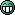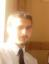# Boxes

2 replies to this topic

### #1Tchernoben

Tchernoben

Casio Fan

•• Members
•• 42 posts
• Location:Nice
• Interests:Calculators, Pc, mangas, warhammer.

• Calculators:
Ti 89 Titanium

Posted 07 April 2006 - 07:10 PM

Hellotooday in history I made this program because I was lazy to listen the teacher (I'm pretty good at History and the teacher gave me his approbation)
It's just a function which allow you to display (in graphic mode) interuption (like windows allerts)
The last section of the program is destinated to test it so if you want use a 'require' please remove it (as the requires on top)
They are fully usables with stylet (you can even move them on the screen)```require("draw")
showgraph()
fullscreen(0)
draw.onbuffer(1)

tch = {}
-- Tchernoben's functions

-- tch.box ( pos_x, pos_y, width, height, s_title, s_body, args )
-- types of arguments :
-- pos_x, pos_y, width, height : unsigned integers ( pos_x, pos_y are the coordinates of the left-top corer )
-- s_title, s_body : strings
-- args : integers {1,2,3} (1="ok", 2="Y/N", 3="Y/N + cancel")
-- EVERY ARGUMENTS MUST BE SET WHEN THE FUNCTION IS CALLED

tch.box = function ( pos_x, pos_y, width, height, s_title, s_body, args )
repeat
pen_x,pen_y = getpen()
if pen_x ~= nil then
if pen_x >= pos_x and pen_x <= pos_x + width and pen_y >= pos_y and pen_y <= pos_y +15 then
draw.rect ( pos_x, pos_y, pos_x + width+1, pos_y + height+1, 0,0,0 )
pos_x, pos_y = pen_x - 0.5*width, pen_y - 7
end
end

draw.rect ( pos_x, pos_y, pos_x + width, pos_y + height, 1,1,0 )
draw.text ( pos_x +5, pos_y +2, s_title, 1,9,2 )
draw.line ( pos_x, pos_y +13, pos_x + width, pos_y +13 )
draw.text ( pos_x +5, pos_y +15, s_body, 1,9,0 )
draw.line ( pos_x +width+1, pos_y +1, pos_x +width+1, pos_y +height+1 )
draw.line ( pos_x +1, pos_y   +height+1,  pos_x +width+1, pos_y +height+1 )

if type(args) == "number" then

if args == 1 then
draw.rect ( pos_x+0.5*width-15, pos_y + 29, pos_x+0.5*width+15, pos_y + 41 )
draw.text ( pos_x+0.5*width-5, pos_y + 31, "OK", 1,9,0 )
if pen_x ~= nil then
if pen_x >= pos_x+0.5*width-15 and pen_x <= pos_x+0.5*width+15 and pen_y >= pos_y +29 and pen_y <= pos_y +41 then
repeat
draw.rect ( pos_x+0.5*width-15, pos_y + 29, pos_x+0.5*width+15, pos_y + 41, 1,1,1 )
draw.update()
until not getpen()
return (0)
end
end
end

if args == 2 then
draw.rect ( pos_x + (1/3)*(width-60), pos_y + 29, pos_x + (1/3)*(width-60)+30, pos_y +41 )
draw.rect ( pos_x + (1/3)*(width-60)+(1/3)*(width-60)+30, pos_y + 29, pos_x + (1/3)*(width-60)+(1/3)*(width-60)+60, pos_y +41 )
draw.text ( pos_x + (1/3)*(width-60)+7, pos_y + 31, "YES", 1,9,0 )
draw.text ( pos_x + (1/3)*(width-60)+(1/3)*(width-60)+40, pos_y + 31, "NO", 1,9,0 )
if pen_x ~= nil then
if pen_x >= pos_x + (1/3)*(width-60) and pen_x <= pos_x + (1/3)*(width-60)+30 and pen_y >= pos_y + 29 and pen_y <= pos_y +41 then
repeat
draw.rect ( pos_x + (1/3)*(width-60), pos_y + 29, pos_x + (1/3)*(width-60)+30, pos_y +41, 1,1,1 )
draw.update()
until not getpen()
return(1)
end
if pen_x >= pos_x + (1/3)*(width-60)+(1/3)*(width-60)+30 and pen_x <= os_x + (1/3)*(width-60)+(1/3)*(width-60)+60 and pen_y >= pos_y + 29 and pen_y <= pos_y +41 then
repeat
draw.rect ( pos_x + (1/3)*(width-60)+(1/3)*(width-60)+30, pos_y + 29, pos_x + (1/3)*(width-60)+(1/3)*(width-60)+60, pos_y +41, 1,1,1 )
draw.update()
until not getpen()
return(2)
end
end
end

if args == 3 then
draw.rect ( pos_x + (1/4)*(width-90), pos_y +29, pos_x + (1/4)*(width-90)+30, pos_y + 41 )
draw.rect ( pos_x + (1/4)*(width-90)+30 + (1/4)*(width-90), pos_y +29, pos_x + (1/4)*(width-90)+30 + (1/4)*(width-90)+30, pos_y +41)
draw.rect ( pos_x + (1/4)*(width-90)+30 + (1/4)*(width-90)+30 + (1/4)*(width-90), pos_y +29, pos_x + (1/4)*(width-90)+30 + (1/4)*(width-90)+30 + (1/4)*(width-90)+30, pos_y +41 )
draw.text ( pos_x + (1/3)*(width-60)-6, pos_y + 31, "YES", 1,9,0 )
draw.text ( pos_x + (1/4)*(width-90)+30 +  (1/4)*(width-90)+10, pos_y + 31, "NO", 1,9,0 )
draw.text ( pos_x + (1/4)*(width-90)+30 + (1/4)*(width-90)+30 + (1/4)*(width-90)+4, pos_y +31, "STOP", 1,9,0 )
if pen_x ~= nil then
if pen_x >= pos_x + (1/4)*(width-90) and pen_x <= pos_x + (1/4)*(width-90)+30 and pen_y >= pos_y +29 and pen_y <= pos_y + 41 then
repeat
draw.rect ( pos_x + (1/4)*(width-90), pos_y +29, pos_x + (1/4)*(width-90)+30, pos_y + 41, 1,1,1 )
draw.update()
until not getpen()
return(1)
end
if pen_x >= pos_x + (1/4)*(width-90)+30 + (1/4)*(width-90) and pen_x <= pos_x + (1/4)*(width-90)+30 + (1/4)*(width-90)+30 and pen_y >= pos_y +29 and pen_y <= pos_y + 41 then
repeat
draw.rect ( pos_x + (1/4)*(width-90)+30 + (1/4)*(width-90), pos_y +29, pos_x + (1/4)*(width-90)+30 + (1/4)*(width-90)+30, pos_y +41, 1,1,1 )
draw.update()
until not getpen()
return(2)
end
if pen_x >= pos_x + (1/4)*(width-90)+30 + (1/4)*(width-90)+30 + (1/4)*(width-90) and pen_x <= pos_x + (1/4)*(width-90)+30 + (1/4)*(width-90)+30 + (1/4)*(width-90)+30 and pen_y >= pos_y +29 and pen_y <= pos_y + 41 then
repeat
draw.rect ( pos_x + (1/4)*(width-90)+30 + (1/4)*(width-90)+30 + (1/4)*(width-90), pos_y +29, pos_x + (1/4)*(width-90)+30 + (1/4)*(width-90)+30 + (1/4)*(width-90)+30, pos_y +41, 1,1,1 )
draw.update()
until not getpen()
return(3)
end
end
end

end

draw.update ()
until false
end

draw.clear ()
for i=1, 3 do
tch.box ( 10,20,120,50, "Question","N' "..i, i )
end```

### #2-Tom-

-Tom-

Casio Freak

•• Members
•• 104 posts
• Location:Poland

• Calculators:

Posted 07 April 2006 - 07:42 PM

Great tool manTry to include keyboard as a way of accepting. I see its Your hobby now to work about GUI, maybe You could write a library which would be useful for everybody... I think Orwell will be busy for some time with debugging so he will not manage to write UI

### #3Tchernoben

Tchernoben

Casio Fan

•• Members
•• 42 posts
• Location:Nice
• Interests:Calculators, Pc, mangas, warhammer.

• Calculators:
Ti 89 Titanium

Posted 09 April 2006 - 12:57 PM

I think I can't create an graphical Input only by Lua and I'm not good enough in Cpp for create a lib

#### 0 user(s) are reading this topic

0 members, 0 guests, 0 anonymous users